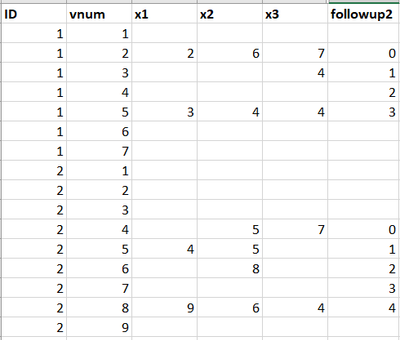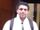## How to line up visit numbers

I have a longitudinal data with multiple outcomes (x1-x3) and visits (vnum). Since people started to get the outcomes of interest measured at different visit, I want to line up the visit numbers to reflect the visits related to my outcome of interest. I want to capture the visits since the first time any of the outcomes was measured until the last time any of them was measured. The variable "followup2" is what I want eventually. Any thoughts?Here's the code for the example data.

``````data have;
input id vnum x1 x2 x3;
cards;
1 1 . . .
1 2 2 6 7
1 3 . . 4
1 4 . . .
1 5 3 4 4
1 6 . . .
1 7 . . .
2 1 . . .
2 2 . . .
2 3 . . .
2 4 . 5 7
2 5 4 5 .
2 6 . 8 .
2 7 . . .
2 8 9 6 4
2 9 . . .
;
run;``````

I was able to get a variable that counts any non-missing visit, but it skipped things like visit 4 for id 1, which I do want to capture too. If it's helpful, I can post those codes too.

1 ACCEPTED SOLUTION

Accepted Solutionsnovinosrin
Tourmaline | Level 20

## Re: How to line up visit numbers

Hi @kivanvan  Please see if this helps-

``````
data have;
input id vnum x1 x2 x3;
cards;
1 1 . . .
1 2 2 6 7
1 3 . . 4
1 4 . . .
1 5 3 4 4
1 6 . . .
1 7 . . .
2 1 . . .
2 2 . . .
2 3 . . .
2 4 . 5 7
2 5 4 5 .
2 6 . 8 .
2 7 . . .
2 8 9 6 4
2 9 . . .
;
run;

data want;
do _n1=1 by 1  until(last.id);
set have;
by id;
if not _n2 and n(of x1-x3) then _n2=_n1;
else if _n2 and n(of x1-x3) then _n3=_n1;
end;
do _n1=1 to _n1;
set have;
if _n1=_n2 then followup2=0;
else followup2=ifn(_n2<_n1<=_n3,sum(followup2, 1),.);
output;
end;
drop _:;
run;
``````
id vnum x1 x2 x3 followup2
1 1 . . . .
1 2 2 6 7 0
1 3 . . 4 1
1 4 . . . 2
1 5 3 4 4 3
1 6 . . . .
1 7 . . . .
2 1 . . . .
2 2 . . . .
2 3 . . . .
2 4 . 5 7 0
2 5 4 5 . 1
2 6 . 8 . 2
2 7 . . . 3
2 8 9 6 4 4
2 9 . . . .

3 REPLIES 3novinosrin
Tourmaline | Level 20

## Re: How to line up visit numbers

Hi @kivanvan  Please see if this helps-

``````
data have;
input id vnum x1 x2 x3;
cards;
1 1 . . .
1 2 2 6 7
1 3 . . 4
1 4 . . .
1 5 3 4 4
1 6 . . .
1 7 . . .
2 1 . . .
2 2 . . .
2 3 . . .
2 4 . 5 7
2 5 4 5 .
2 6 . 8 .
2 7 . . .
2 8 9 6 4
2 9 . . .
;
run;

data want;
do _n1=1 by 1  until(last.id);
set have;
by id;
if not _n2 and n(of x1-x3) then _n2=_n1;
else if _n2 and n(of x1-x3) then _n3=_n1;
end;
do _n1=1 to _n1;
set have;
if _n1=_n2 then followup2=0;
else followup2=ifn(_n2<_n1<=_n3,sum(followup2, 1),.);
output;
end;
drop _:;
run;
``````
id vnum x1 x2 x3 followup2
1 1 . . . .
1 2 2 6 7 0
1 3 . . 4 1
1 4 . . . 2
1 5 3 4 4 3
1 6 . . . .
1 7 . . . .
2 1 . . . .
2 2 . . . .
2 3 . . . .
2 4 . 5 7 0
2 5 4 5 . 1
2 6 . 8 . 2
2 7 . . . 3
2 8 9 6 4 4
2 9 . . . .novinosrin
Tourmaline | Level 20

## Re: How to line up visit numbers

Or simpler-

``````
data have;
input id vnum x1 x2 x3;
cards;
1 1 . . .
1 2 2 6 7
1 3 . . 4
1 4 . . .
1 5 3 4 4
1 6 . . .
1 7 . . .
2 1 . . .
2 2 . . .
2 3 . . .
2 4 . 5 7
2 5 4 5 .
2 6 . 8 .
2 7 . . .
2 8 9 6 4
2 9 . . .
;
run;

proc sql;
create view temp as
select id, min(case when n(x1,x2,x3)>0 then vnum else . end) as start,
max(case when n(x1,x2,x3)>0 then vnum else . end) as stop
from have
group by id;
quit;

data want;
merge have temp;
by id;
if first.id then followup2=.;
retain followup2;
if vnum=start then followup2=0;
else followup2=ifn(start<vnum<=stop,sum(followup2,1),.);
drop start stop;
run;``````

## Re: How to line up visit numbers

It works perfect! Thank you! @novinosrin

Discussion stats
• 3 replies
• 389 views
• 0 likes
• 2 in conversation